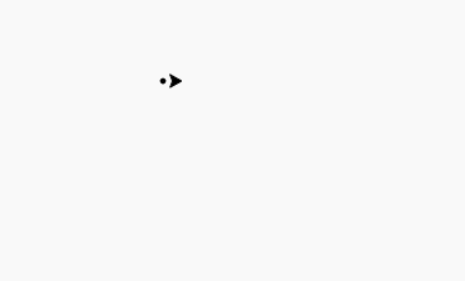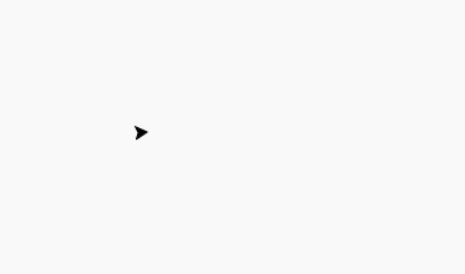GeeksforGeeks App
Open AppBrowser
Continue

# Draw Dot Patterns Using Turtle in Python

Prerequisite: Turtle Programming Basics

Turtle is an inbuilt module in Python. It provides drawing using a screen (cardboard) and turtle (pen). To draw something on the screen, we need to move the turtle (pen). To move turtle, there are some functions i.e forward(), backward(), etc.

#### 1) Draw Dot Square

Following steps are used :

• Import turtle
• Make turtle
• Define a function to draw a square with dots
• Call that function
• Hide the turtle.

Below is the implementation :

## Python3

 `# import package and making object``import` `turtle `` ` ` ` `pen ``=` `turtle.Turtle()`` ` `# method to draw square with dots``# space --> distance between dots``# x     --> side of square``def` `draw(space,x):``  ``for` `i ``in` `range``(x):``    ``for` `j ``in` `range``(x):``       ` `        ``# dot``        ``pen.dot()``         ` `        ``# distance for another dot``        ``pen.forward(space)``    ``pen.backward(space``*``x)``     ` `    ``# direction``    ``pen.right(``90``)``    ``pen.forward(space)``    ``pen.left(``90``)`` ` `# Main Section``pen.penup()``draw(``10``,``8``)`` ` `# hide the turtle``pen.hideturtle()`

Output :#### 2) Draw Dot Rectangle

Following steps are used :

• Import turtle
• Make turtle
• Define a function to draw a rectangle with dots
• Call that function
• Hide the turtle.

Below is the implementation :

## Python3

 `# import package and making object``import` `turtle `` ` ` ` `pen ``=` `turtle.Turtle()`` ` `# method to draw rectangle with dots``# space --> distance between dots``# x     --> height of rectangle``# y     --> width of rectangle``def` `draw(space,x,y):``  ``for` `i ``in` `range``(x):``    ``for` `j ``in` `range``(y):``       ` `        ``# dot``        ``pen.dot()``         ` `        ``# distance for another dot``        ``pen.forward(space)``    ``pen.backward(space``*``y)``     ` `    ``# direction``    ``pen.right(``90``)``    ``pen.forward(space)``    ``pen.left(``90``)`` ` `# Main Section``pen.penup()``draw(``10``,``5``,``12``)`` ` `# hide the turtle``pen.hideturtle()`

Output :#### 3) Draw Dot Diamond:

Following steps are used :

• Import turtle
• Make turtle
• Define a function to draw a diamond with dots
• Call that function
• Hide the turtle.

Below is the implementation :

## Python3

 `# import package and making object``import` `turtle `` ` ` ` `pen ``=` `turtle.Turtle()`` ` `# method to draw diamond with dots``# space --> distance between dots``# x     --> side of diamond``def` `draw(space,x):``  ``for` `i ``in` `range``(x):``    ``for` `j ``in` `range``(x):``         ` `        ``# dot``        ``pen.dot()``         ` `        ``# distance for another dot``        ``pen.forward(space)``    ``pen.backward(space``*``x)``     ` `    ``# direction``    ``pen.right(``90``)``    ``pen.forward(space)``    ``pen.left(``90``)`` ` `# Main Section``pen.penup()`` ` `# direction to form diamond``pen.left(``45``)``draw(``10``,``8``)`` ` `# hide the turtle``pen.hideturtle()`

Output :My Personal Notes arrow_drop_up